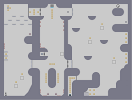### Support BeamHover over the thumbnail for a full-size version.

Author scarlet action author:scarlet playable unrated 2007-06-21 2007-06-21 4 more votes required for a rating. \$Support Beam#scarlet#none#000000000000000000000000000000000000000000000000000000000000000000000000000000000000000000000000000000000000000000000000000;<0000000000003100<00001100;<00;<00GK1100111111111110011111111001111111111100:111111100=0000000101000000006=0000000000:0=0000000000000000000000000;111<000000000000000000:111=00<0000000000000000000000100<00000000000000000071001111111111<00;111111100=000000:111001100000000000<000000000110;111000000=000000000:=01111000000000000000000011110000000000000<0000011=0000<000000000=000001100000=0000000000000;1110000000000000000000:11=00000000000<0000000000000000000000=0000000000000000000000000000000000;10;<00;<00000000000000110110011000000000000;1110:=0011000;<0000;111111000001100011000;1111111|5^36,564!0^348,468!0^348,480!0^348,492!0^300,384!0^312,384!0^324,384!7^132,108,3!7^84,108,3!7^60,132,3!7^108,132,3!7^84,156,3!7^36,156,3!7^132,156,3!7^156,132,3!1^372,324!7^420,396,0!7^420,372,0!9^756,132,0,0,11,13,1,-1,0!0^756,372!0^756,348!0^756,324!0^756,252!0^756,228!0^756,204!1^684,372!1^540,252!7^36,348,1!7^36,324,1!7^36,300,1!12^24,288!12^24,276!12^24,264!7^180,156,3!3^180,204!8^312,36,1!3^288,564!3^336,564!12^276,576!12^264,576!12^240,552!12^240,540!12^360,576!12^348,576!12^384,552!12^384,540!0^348,456!0^348,444!0^276,444!0^276,456!0^276,468!0^276,480!0^276,492!11^516,564,252,324!9^48,48,0,0,16,15,1,0,0!9^48,48,0,0,16,16,1,0,0!1^132,468!1^96,516!7^180,372,0!7^180,396,0!7^444,84,2!7^444,60,2!0^444,468!9^456,468,0,0,19,19,0,-1,0!0^132,444!0^96,492!0^684,348!0^540,228!0^612,144!1^612,168!0^228,72!0^180,72!0^312,60!0^312,72!0^312,84!0^312,96!0^312,108!0^540,564!0^540,540!0^564,540!0^564,564!0^36,324!0^36,348!0^48,60!0^60,48!0^48,36!0^36,NaN!12^264,288!12^252,288!12^240,288!12^240,276!12^240,264!0^432,468!0^420,468!0^420,480!0^420,504!0^420,492!0^420,516!0^420,528!0^420,540!0^420,552!12^420,NaN!6^708,36,1,0,1,2!4^420,570,1!9^168,84,0,0,7,3,0,-1,0!9^168,60,0,0,7,2,0,-1,0!9^408,72,0,0,10,3,1,-1,0!9^408,72,0,0,10,2,1,-1,0!0^204,72!0^204,60!0^204,84!12^384,132!12^384,156!12^384,180!12^240,132!12^240,156!12^240,NaN# Infiltrate the base! Not as hard as a lot of other maps here, but still tough for me. Have fun!